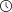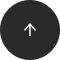- 8 minutes read

# 15 Python Interview Questions for Data Science Jobs

Looking for a data science job? Then you've probably noticed that most positions require applicants to have some level of Python programming skills. But how are they going to test this? What are they going to ask? Let's prepare you for some interview questions!

### Why Do Data Scientists Need Python?

Data science goes beyond simple data analysis and requires that you be able to work with more advanced tools. Thus, if you work with big data and need to perform complex computations or create aesthetically pleasing and interactive plots, Python is one of the most efficient solutions out there.

Python's readability and simple syntax make it relatively easy to learn, even for non-programmers. Moreover, Python has plenty of data analysis libraries that make your work easier.

Of course, Python requirements for data scientists are different from those for software engineers and developers. Data scientists should be comfortable with basic Python syntax, built-in data types, and the most popular libraries for data analysis. These are the topics that are usually covered in the Python interview questions for data science.

### Sample Python Interview Questions and Answers

We've selected 15 Python interview questions that are most commonly asked by employers during interviews for entry-level data science positions. This set covers some Python coding interview questions and also some non-technical questions. Be prepared to explain some specific features of the Python language and also demonstrate that you can explore, visualize, and model a given data set.

#### 1. What are the data types used in Python?

Python has the following built-in data types:

• Number (float, integer)
• String
• Tuple
• List
• Set
• Dictionary

Numbers, strings, and tuples are immutable data types, meaning they cannot be modified during runtime. Lists, sets, and dictionaries are mutable, which means they can be modified during runtime.

#### 2. Explain the difference between lists and tuples.

Both lists and tuples are made up of elements, which are values of any Python data type. However, these data types have a number of differences:

• Lists are mutable, while tuples are immutable.
• Lists are created with square brackets (e.g., my_list = [a, b, c]), while tuples are enclosed in parentheses (e.g., my_tuple = (a, b, c)).
• Lists are slower than tuples.

#### 3. What is a Python dictionary?

A dictionary is one of the built-in data types in Python. It defines an unordered mapping of unique keys to values. Dictionaries are indexed by keys, and the values can be any valid Python data type (even a user-defined class). Notably, dictionaries are mutable, which means they can be modified. A dictionary is created with curly braces and indexed using the square bracket notation.

Here's an example:

```my_dict = {'name': 'Hugh Jackman', 'age': 50, 'films': ['Logan', 'Deadpool 2', 'The Front Runner']}
my_dict['age']
```

Here, the keys include `name`, `age`, and `films`. As you can see, the corresponding values can be of different data types, including numbers, strings, and lists. Notice how the value 50 is accessed via the corresponding key age.

#### 4. What are lambda functions?

Lambda functions are anonymous functions in Python. They're very helpful when you need to define a function that's very short and consists of only one expression. So, instead of formally defining the small function with a specific name, body, and return statement, you can write everything in one short line of code using a lambda function.

Here's an example of how lambda functions are defined and used:

```(lambda x, y, z: (x+y) ** z)(3,2,2)
```
`25`

In this example, we've defined an anonymous function that has three arguments and takes the sum of the first two arguments (x and y) to the power of the third argument (z). As you can see, the syntax of a lambda function is much more concise than that of a standard function.

#### 5. Explain list comprehensions and how they're used in Python.

List comprehensions provide a concise way to create lists.

A list is traditionally created using square brackets. But with a list comprehension, these brackets contain an expression followed by a for clause and then if clauses, when necessary. Evaluating the given expression in the context of these for and if clauses produces a list.

It's best explained with an example:

```old_list = [1, 0, -2, 4, -3]
new_list = [x**2 for x in old_list if x > 0]
print(new_list)
```
`[1,16]`

Here, we're creating a new list by taking the elements of the old list to the power of 2, but only for the elements that are strictly positive. The list comprehension allows us to solve this task in just one line of code.

#### 6. What is a negative index, and how is it used in Python?

A negative index is used in Python to index a list, string, or any other container class in reverse order (from the end). Thus, [-1] refers to the last element, [-2] refers to the second-to-last element, and so on.

Here are two examples:

```list = [2, 5, 4, 7, 5, 6, 9]
print (list[-1])```
`9`
```text = "I love data science"
print (text[-3])```
`n`

#### 7. Name some well-known Python data analysis libraries.

If you're doing data analysis with Python, you're likely going to use:

• NumPy
• Pandas
• Matplotlib
• Seaborn
• SciKit

These libraries will help you work with arrays and DataFrames, build professional-looking plots, and run machine learning models.

If you're looking to review your knowledge of the pandas and Matplotlib libraries, check out this Introduction to Python for Data Science course.

#### 8. What is pandas?

Pandas is a Python open-source library that provides high-performance and flexible data structures and data analysis tools that make working with relational or labeled data both easy and intuitive.

#### 9. Write Python code to create an employees DataFrame from the "HR.csv" file.

We can create a DataFrame from a CSV file by using the `read_csv()` function from the `pandas` library. By convention, pandas is imported as `pd`. So the code is simply the following:

`import pandas as pd`
`emloyees = pd.read_csv('HR.csv')`

#### 10. What is the default missing value marker in pandas, and how can you detect all missing values in a DataFrame?

In pandas, the default missing value marker is `NaN`.

You can detect all missing values in a DataFrame by using the `isna()` function from the `pandas` library:

`employees.isna()`
ID Department Age
0 False False False
1 False False True
2 False False False
3 False True False
4 False False True

To explore the composition of a column, including how many missing values it has, use the info() function; it shows the number of non-null entries in each column together with the total number of entries in that column (the difference, of course, is the number of missing values!).

`emploees.info()`
```
RangeIndex: 100 entries, 0 to 99
Data columns (totas 3 columns):
ID	100 non-null int64
Department	92 non-null object
Age	94 non-null float64
dtypes: float64(1), int64(1), object(1)
memory usage: 2.4+ KB```

### 11. Write Python code to select the Department and Age columns from the employees DataFrame.

You can select multiple columns from a DataFrame by passing in their names to double square brackets:

```employees[['Department', 'Age']]
```
Departmentage Age
0 Sales 34.0
1 Finance NaN
2 HR 25.0
3 NaN 46.0
4 Administration NaN
5 Sales 56.0

#### 12. Add a new column named Active to the employees DataFrame with all 1s to indicate each employee's active employment status.

You can create a new column with a particular value for all entries by simply assigning this value to the whole column:

`employees['Active'] = 1`
`employees.head()`
ID Departmentage Age Active
0 1 Sales 34.0 1
1 2 Finance NaN 1
2 3 HR 25.0 1
3 4 NaN 46.0 1
4 5 Administration NaN 1

#### 13. Write Python code to plot the distribution of employees by age.

To plot the distribution of employees by age, you'll simply need to create a histogram from the Age column of the `employees` DataFrame. This can be done by calling the `hist()` function on the selected column:

```employees['Age'].hist()
```#### 14. What libraries do data scientists use to plot data in Python?

Matplotlib is the main library used for plotting data in Python. However, the plots created with this library need lots of fine-tuning to look shiny and professional. For that reason, many data scientists prefer Seaborn, which allows you to create appealing and meaningful plots with only one line of code.

#### 15. What Python IDEs are the most popular in data science?

Granted, you won't be asked this exact question, but it's likely that your prospective employer will still want to get a better sense of how you work with Python and what exposure you have to the language.

In that case, it's good to know that Jupyter Notebook, PyCharm, and Spyder are all good Python IDEs, but Jupyter Notebook is arguably the best for data science.

Interested in exploring some other Python IDEs for data science? Check out this article.

### Summary

We hope these Q&As will help you feel confident during your next interview for that entry-level data science position you've always wanted. Of course, keep in mind that these are only some of the most popular Python interview questions asked at the entry level; you may be asked some other technical questions, too.

If you want to gain more comprehensive knowledge of Python for data science, check out the Introduction to Python for Data Science, which covers most of the questions mentioned in this article—and much more.